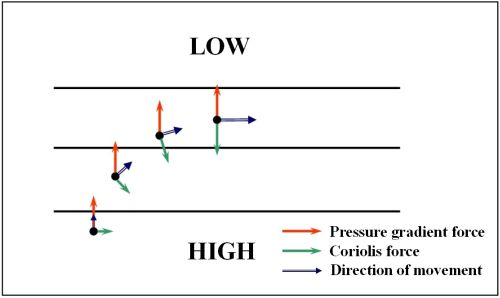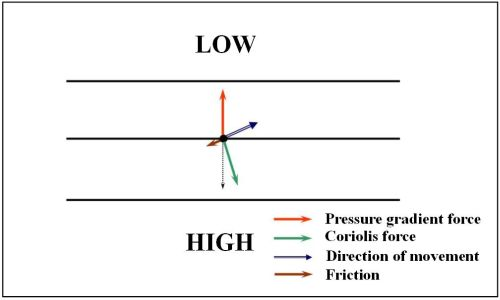# Geostrophic WindEducational Resources

Geostrophic Wind

Differential heating on the Earth's surface causes variation in air temperatures at different places.  Warmer air expands and brings lower air density and pressure, while the surrounding cooler air has a higher air density and pressure.  The difference in air pressure between two places is called the pressure gradient.  Winds are generated from the driving force derived from the pressure gradient.  The tighter the pressure gradient, the larger will be the driving force, and hence the stronger the winds will be.

In general, wind blows from high pressure to low pressure.  In reality, besides air pressure, the Earth's rotation and the grounds friction also influence the wind direction.  Relative to an observer in the northern hemisphere, a moving air mass will be deflected to the right due to the Earths rotation.  Why would this happen?

In the northern hemisphere, as the Earth rotates, air moves together with the Earth's surface from west to east.  Let's take the example of an air parcel moving from A to B as shown in Figure 1.  Owing to the Earth's rotation, point A will move to position A' and the air mass will move to position C instead of B'.  This is because the eastward moving speed of point A is faster than the speed of point B at higher latitude.  Therefore, relative to an observer at point A', the air mass will be deflected to the right.Figure 1  Deflection of a moving air mass on the Earth's surface (diagram not to scale)

The apparent deflection of a moving air mass caused by the Earth's rotation is called the Coriolis effect.  In meteorology, the effect of the Earth's rotation is represented by a Coriolis force acting on the air mass, deflecting it to the right in the northern hemisphere and to the left in the southern hemisphere.  The direction of the Coriolis force is perpendicular to the direction of air movement.  The Coriolis force is greater at higher latitudes and smaller near the equator.

Now, we can explain the relationship between the wind direction and orientation of isobars on a weather chart.  Figure 2 shows an air mass moving from high pressure to low pressure under the pressure gradient force in the northern hemisphere.  As the air mass starts to move, it is deflected to the right by the Coriolis force.  The deflection increases until the Coriolis force is balanced by the pressure gradient force.  At this point, the wind will be blowing parallel to the isobars.  When this happens, the wind is referred to as the "geostrophic wind".  From Figure 2, we have a simple relationship between the wind direction and the pressure distribution in the northern hemisphere: if we stand with our back towards the wind, the pressure to the left is lower than that to the right.Figure 2      Schematic diagram of geostrophic wind formation

Geostrophic wind is an ideal situation.  In practice, we have to consider the effect of friction between the air and the ground.  When the pressure gradient force, frictional force and Coriolis force are in equilibrium, the wind direction will be pointing at a small angle towards the low pressure side, as shown in Figure 3.Figure 3    The balance between the pressure gradient force and the resultant (dotted line) of Coriolis force and frictional force

As the friction acting on an air parcel is smaller over the sea than the land, geostrophic winds in general align better with the actual winds over the ocean.